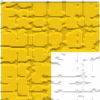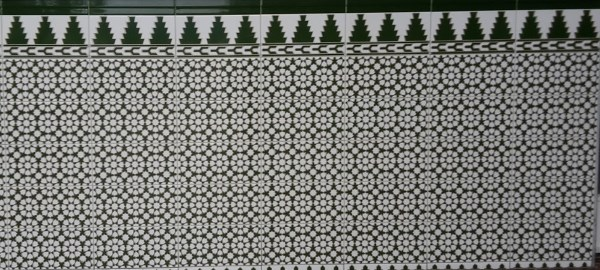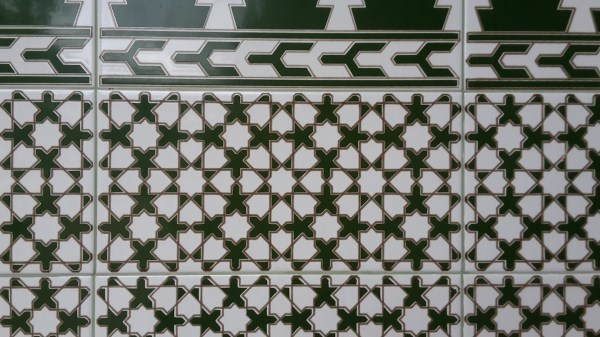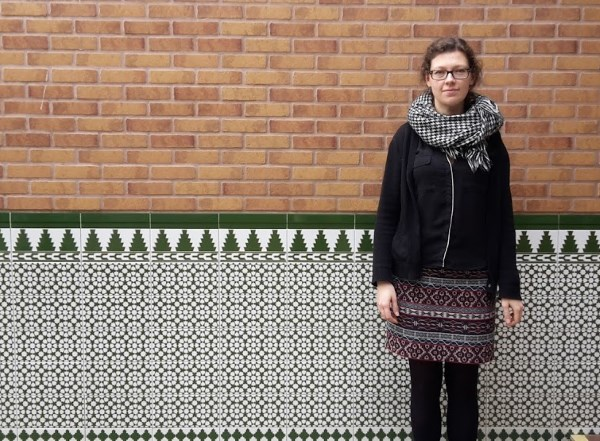#### You may also like### Triominoes

A triomino is a flat L shape made from 3 square tiles. A chess board is marked into squares the same size as the tiles and just one square, anywhere on the board, is coloured red. Can you cover the board with trionimoes so that only the square is exposed?### LOGO Challenge 5 - Patch

Using LOGO, can you construct elegant procedures that will draw this family of 'floor coverings'?### LOGO Challenge - Triangles-squares-stars

Can you recreate these designs? What are the basic units? What movement is required between each unit? Some elegant use of procedures will help - variables not essential.

# Gibraltar Geometry

### Why do this problem?

In this problem, we present a photograph that has lots of intriguing patterns that we hope will provoke students' curiosity and prompt them to ask mathematical questions.

The main mathematical themes are tiling, tessellation and symmetry, but there is also an opportunity to think about area and estimation by considering how many tiles would be needed to cover a particular area.

### Possible approach

Show the first picture:Give students a little while to look at it, and then share the closeup showing detail of the tiles:Give students a bit more time to look at it, and then invite them to share mathematical questions that occur to them.
Here are some that might arise:
• What geometric shapes are there in the tiles?
• What angles can you work out?
• How many squares are there?
• How many eight-pointed stars?
• How big are the tiles?
• How many rectangular tiles would you need to tile your classroom?
Students could choose a question to explore, or you could guide them towards a particular topic. This worksheet contains a black-and-white closeup of the tile together with the questions above.

The third picture gives some context by positioning Becky, who is 1.7m tall, in front of the tiles. This opens up the possibility of estimating the size of the tiles, and the number that would be needed to cover a given area.### Key questions

What can you see?
What mathematical questions do you want to explore?

### Possible extension

There are lots of suitable follow-up questions in the Angles, Polygons and Geometrical Proof Short Problems Collection.

### Possible support

Students could use squared paper or origami paper to draw or fold simpler patterns of their own inspired by the designs in the pictures.